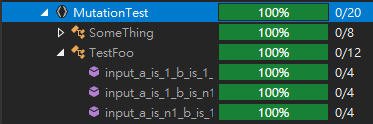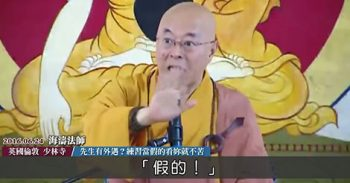### Test - 變異(Mutation)測試之你的測試到底是寫爽的，還是有效的?public class SomeThing
{
public int Foo(int a, int b)
{
var c = 0;
if (a > 0 && b > 0)
c = b;
return c;
}
}


[TestClass]
public class TestFoo
{
[TestMethod]
public void input_a_is_1_b_is_1_Should_1()
{
var someThing = new SomeThing();
Assert.AreEqual(1, someThing.Foo(1, 1));
}

[TestMethod]
public void input_a_is_1_b_is_n1_should_be_0()
{
var someThing = new SomeThing();
Assert.AreEqual(0, someThing.Foo(1, -1));
}

[TestMethod]
public void input_a_is_n1_b_is_1_should_be_0()
{
var someThing = new SomeThing();
Assert.AreEqual(0, someThing.Foo(-1, 1));
}

}public class SomeThing
{
public int Foo(int a, int b)
{
var c = 0;
if (a > 0 && b >= 0)
c = b;
return c;
}
}


• ## Code Coverage 是假的• 條件邊界的變異
• 反向條件的變異
• 移除條件的變異
• 遞增、遞減的變異
• 常數的變異
• 返回值的變異
• 移除程式碼的變異

## 反向條件的變異

public class SomeThing
{
public int Foo(int a, int b)
{
var c = 0;
if (!(a > 0 && b >= 0))
c = b;
return c;
}
}

## 移除條件的變異

public class SomeThing
{
public int Foo(int a, int b)
{
var c = 0;
c = b;
return c;
}
}

## 返回值的變異

public class SomeThing
{
public int Foo(int a, int b)
{
var c = 0;
if (a > 0 && b > 0)
c = b;
return 0;
}
}


## 移除程式碼的變異

public class SomeThing
{
public int Foo(int a, int b)
{
var c = 0;
return c;
}
}


## 結語

• 這些被移除的程式碼根本不重要，有跟沒有一樣
• 你的測試程式碼的有效性不足夠，所以要加新的測試

• ### 確定異變程式碼過後，執行測試應該要是失敗的

PS: 工具找出來的變異測試失敗，不一定是真的失敗，有些情境上是工具無法辨別的，不要被工具限制住了